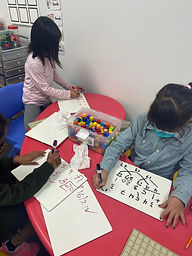## Ms. Hiral

### Target 1​

###### Lesson Type:

New

Measurment

:

Techniques and Formulas

Find the perimeter of a given shape.

###### 1:

Define perimeter.

###### 2:

Understand that perimeter is the distance around a figure.

###### 3:

Understand that perimeter can be found by adding the lengths of the sides of a figure.

###### 4:

See a link between finding the perimeter of rectangles and multiplication.

###### 5:

Understand that figures with the same perimeter can look different.

2nd

###### Vocabulary:

Perimeter, Distance, Length, Width

Activities:

Students found perimeter of different shapes.

Students found the perimeter of rectangle and square using multiplication.

Students created their own shapes on array boards and calcumated their perimeters.

Students drew figures and labeled sides then students figure out the perimeters of their classmates figures.### Home Exploration

###### Guiding Questions:## Absent Students:

### Target 2

:

###### 1:

Use tally marks to collect and track quantitative data.

###### 2:

Looking at a picture where information is chaotic or spread out, count to gather data to answer questions like, how many are there? Are there more ___ or ___? How many more ___ are there than ___?

###### 3:

Understand that data can be read more quickly in graph form.

2nd

###### Vocabulary:

Tally, Quantitative, Data, Graph

Activities:

Students discussed the different graphs they have experience with shared what they look like with each other.

Students then rolled constructed a bar graph by rolling 10 sided dice and seeing how many times they rolled each number with tally marks. Students then used post-its to create the bars on the bar graph (number of post-its corresponded to the tally marks per number).

Students then graphed the same data onto a line graph.

Students then discussed the way each graph presented the same information and which was easier to read.### Home Exploration

###### Guiding Questions:### Target 3

:

###### Vocabulary:

Activities:### Home Exploration